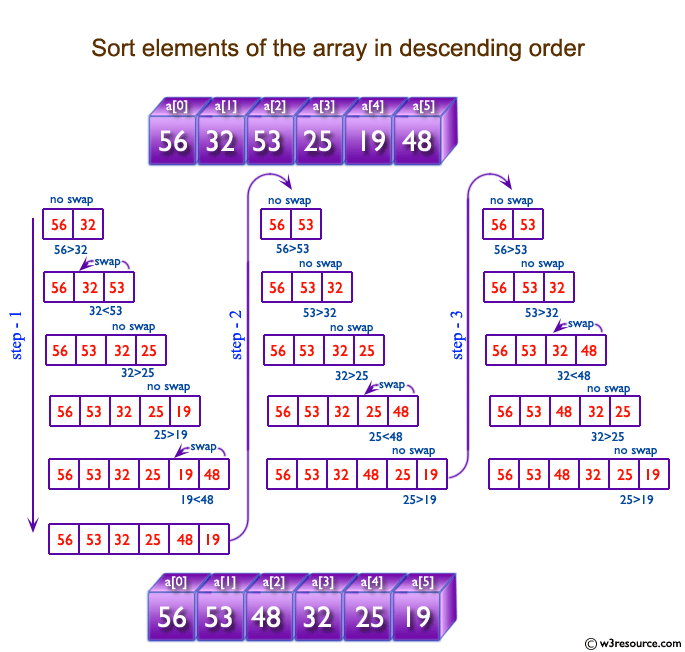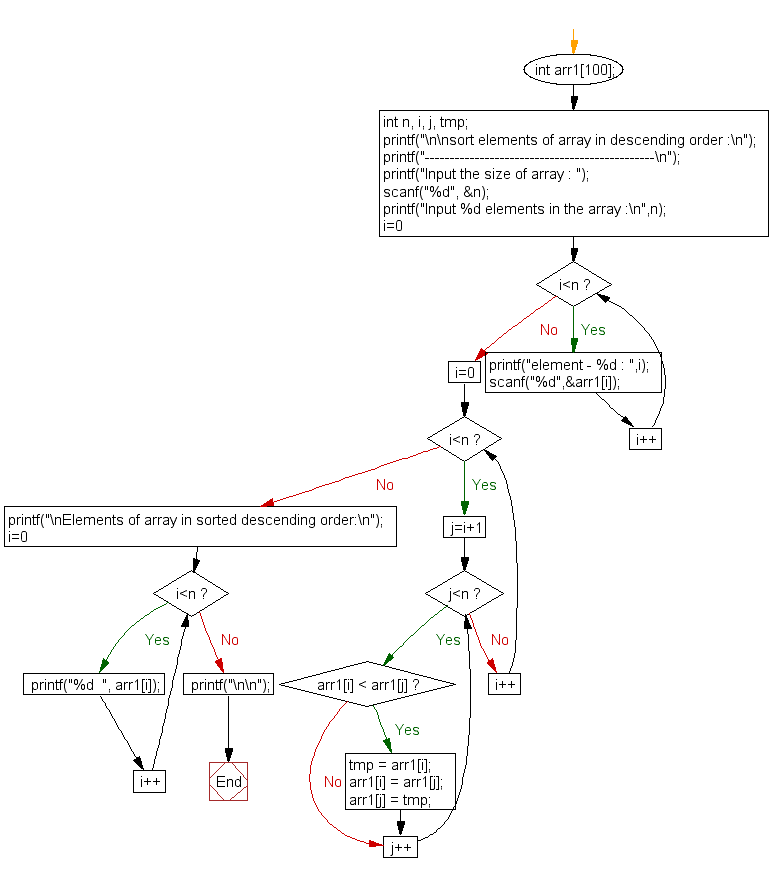﻿ C exercises: Sort elements of an array in descending order - w3resource# C Exercises: Sort elements of an array in descending order

## C Array: Exercise-12 with Solution

Write a program in C to sort elements of an array in descending order.

Pictorial Presentation:Sample Solution:

C Code:

``````#include <stdio.h>

void main()
{
int arr1;
int n, i, j, tmp;

printf("\n\nsort elements of array in descending order :\n");
printf("----------------------------------------------\n");

printf("Input the size of array : ");
scanf("%d", &n);

printf("Input %d elements in the array :\n",n);
for(i=0;i<n;i++)
{
printf("element - %d : ",i);
scanf("%d",&arr1[i]);
}
for(i=0; i<n; i++)
{
for(j=i+1; j<n; j++)
{
if(arr1[i] < arr1[j])
{
tmp = arr1[i];
arr1[i] = arr1[j];
arr1[j] = tmp;
}
}
}

printf("\nElements of array is sorted in descending order:\n");
for(i=0; i<n; i++)
{
printf("%d  ", arr1[i]);
}
printf("\n\n");
}
```
```

Sample Output:

```sort elements of array in descending order :
----------------------------------------------
Input the size of array : 3
Input 3 elements in the array :
element - 0 : 5
element - 1 : 9
element - 2 : 1

Elements of array is sorted in descending order:
9  5  1
```

Flowchart:C Programming Code Editor:

Improve this sample solution and post your code through Disqus.

What is the difficulty level of this exercise?

﻿